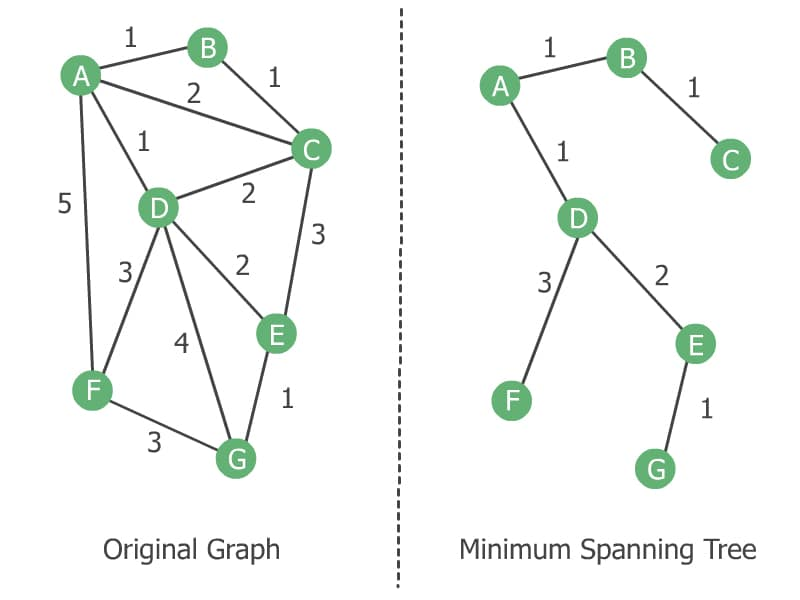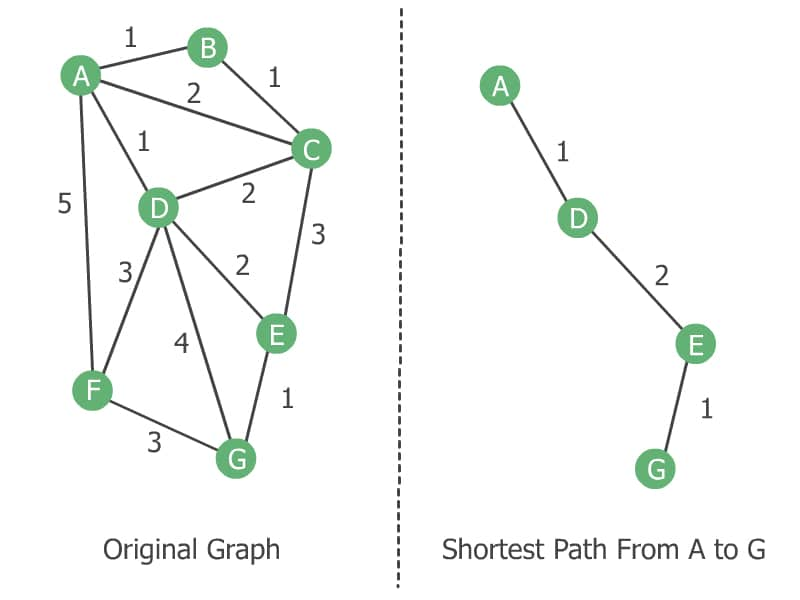## 1. Introduction

Prim’s algorithm and Dijkstra’s algorithm are both famous standard graph algorithms. In this quick tutorial, we’ll discuss the difference between Prim’s and Dijkstra’s algorithms.

Before we proceed, let’s take a look at the two key definitions: minimum spanning tree and shortest path.

## 2. Minimum Spanning Tree

Given an undirected weighted graph, a minimum spanning tree (MST) is a subgraph that connects all the vertices with the lowest possible sum of its edge weights.

Let’s depict it with an example:On the right side, we have the MST of the graph on the left. Note that an MST always contains all vertices of the original graph.

## 3. Shortest Path

Given a weighted graph, the shortest path (or geodesic path) between two vertices is a path with the lowest possible sum of edge weights.

Let’s find the shortest path from A to G in our graph:As you can see in the above image, a shortest-path tree may not contain all vertices of the original graph.

## 4. Comparing Prim’s and Dijkstra’s Algorithms

In the computation aspect, Prim’s and Dijkstra’s algorithms have three main differences:

1. Dijkstra’s algorithm finds the shortest path, but Prim’s algorithm finds the MST
2. Dijkstra’s algorithm can work on both directed and undirected graphs, but Prim’s algorithm only works on undirected graphs
3. Prim’s algorithm can handle negative edge weights, but Dijkstra’s algorithm may fail to accurately compute distances if at least one negative edge weight exists

In practice, Dijkstra’s algorithm is used when we want to save time and fuel traveling from one point to another. Prim’s algorithm, on the other hand, is used when we want to minimize material costs in constructing roads that connect multiple points to each other.

## 5. Conclusion

In this tutorial, we discussed the difference between Prim’s and Dijkstra’s algorithms. Detailed explanations and implementations are available in our articles about Prim’s and Dijkstra’s, respectively.

1 Comment
Inline Feedbacks